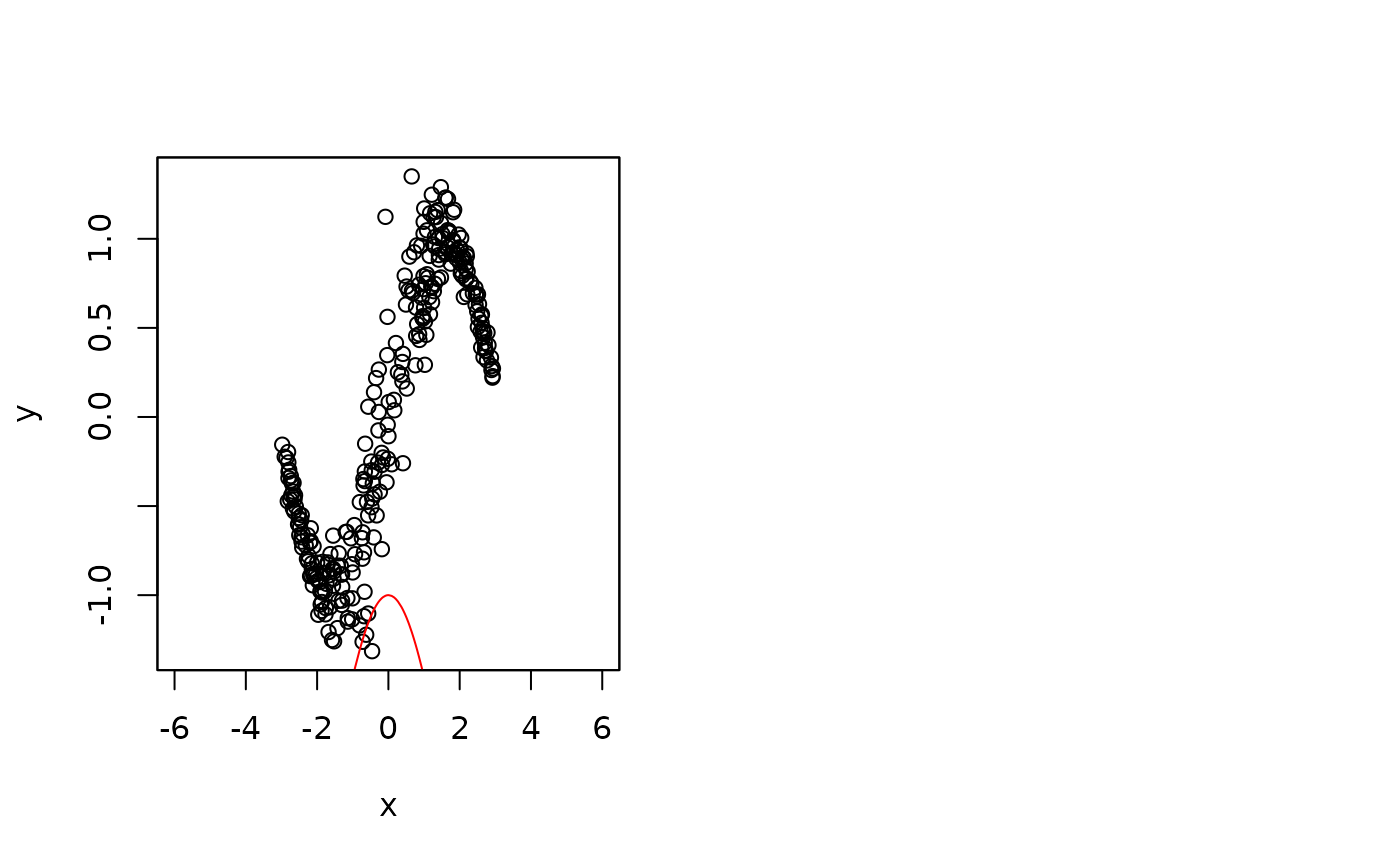This function interfaces keras infrastructures for high-level neural networks. The function can be used as a standalone model fitting engine such as bamlss or as an on top model engine to capture special features in the data that could not be captures by other model fitting engines.

## Deep distributional neural net.
learning_rate = 0.01,
epochs = 100, batch_size = NULL,
nlayers = 2, units = 100, activation = "relu",
l1 = NULL, l2 = NULL,
validation_split = 0.2, early_stopping = TRUE, patience = 50,
verbose = TRUE, ...)

## Predict method.
# S3 method for ddnn
predict(object, newdata,
model = NULL, type = c("link", "parameter"),
drop = TRUE, ...)

## CV method for optimizing
## the number of epochs using
## the CRPS.
cv_ddnn(formula, data, folds = 10,
min_epochs = 300, max_epochs = 400,
interval = c(-Inf, Inf), ...)

## Arguments

object

An object of class "bamlss" or a bamlss.formula.

optimizer

Character or call to optimizer functions to be used within fit. For character, options are: "adam" "sgd", "rmsprop", "adagrad", "adadelta", "adamax", "adam". The default is optimizer_rmsprop with learning rate set to 1e-04.

learning_rate

The learning rate of the optimizer.

epochs

Number of times to iterate over the training data arrays, see fit.

batch_size

Number of samples per gradient update, see fit.

nlayers

Number of hidden layers.

units

Number of nodes per hidden layer, can be a vector.

activation

Activation functions used for the hidden layers, can be a vector.

l1

Shrinkage parameter for L1 penalty.

l2

Shrinkage parameter for L2 penalty.

validation_split

Proportion of data that should be used for validation.

early_stopping

Logical, should early stopping of the optimizer be applied?

patience

Integer, number of iterations the optimizer waits until early stopping is applied after changes get small in validation data set.

verbose

Print information during runtime of the algorithm.

newdata

A list or data.frame that should be used for prediction.

model

Character or integer specifying for which distributional parameter predictions should be computed.

type

If type = "link" the predictor of the corresponding model is returned. If type = "parameter" predictions on the distributional parameter scale are returned.

drop

If predictions for only one model are returned, the list structure is dropped.

formula

The model formula.

data

The data used for estimation.

folds

The number of folds that should be generated.

min_epochs, max_epochs

Defines the minimum and maximum epochs thet should be used.

interval

Response interval, see function CRPS.

...

Arguments passed to bamlss.frame.

## Details

The default keras model is a sequential model with two hidden layers with "relu" activation function and 100 units in each layer. Between each layer is a dropout layer with 0.1 dropout rate.

## Value

For function ddnn() an object of class "ddnn". Note that extractor functions fitted and residuals.bamlss can be applied. For function predict.ddnn() a list or vector of predicted values.

## WARNINGS

The deep learning infrastructure is experimental!

bamlss.frame, bamlss

## Examples

if (FALSE) ## Simulate data.
set.seed(123)
n <- 300
x <- runif(n, -3, 3)
fsigma <- -2 + cos(x)
y <- sin(x) + rnorm(n, sd = exp(fsigma))

## Setup model formula.
f <- list(
y ~ x,
sigma ~ x
)

## Fit neural network.
library("keras")
#>
#> Attaching package: ‘keras’
#> The following object is masked from ‘package:rjags’:
#>
b <- ddnn(f, epochs = 2000)

## Plot estimated functions.
par(mfrow = c(1, 2))
plot(x, y)
plot2d(fitted(b)$mu ~ x, add = TRUE) #> Error in fitted(b): object 'b' not found plot2d(fitted(b)$sigma ~ x,
ylim = range(c(fitted(b)$sigma, fsigma))) #> Error in fitted(b): object 'b' not found plot2d(fsigma ~ x, add = TRUE, col.lines = "red") ## Predict with newdata. nd <- data.frame(x = seq(-6, 6, length = 100)) nd$p <- predict(b, newdata = nd, type = "link")

par(mfrow = c(1, 2))plot(x, y, xlim = c(-6, 6), ylim = range(c(nd$p$mu, y)))
plot2d(p$mu ~ x, data = nd, add = TRUE) #> Error in h(simpleError(msg, call)): error in evaluating the argument 'x' in selecting a method for function 'as.matrix': object 'p' not found plot2d(p$sigma ~ x, data = nd,
ylim = range(c(nd$p$sigma, fsigma)))
#> Error in h(simpleError(msg, call)): error in evaluating the argument 'x' in selecting a method for function 'as.matrix': object 'p' not found
plot2d(fsigma ~ x, add = TRUE, col.lines = "red")

## Plot quantile residuals.
e <- residuals(b)